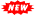# Mathematics Practical Exam-Maths Lab Files

2

Maths Lab-Maths Practical Exam Files For 2024

Lab Manual for Second Year  Maths Practical Exam  2024  by SCERT

Maths Practical Exam Guidelines for 2024 by SCERT

Maths Lab-Maths Practical Exam Files 2023

Maths Practical Exam Guidelines for 2023 by SCERT

Maths Lab Manual for Practical Exam  2023

Applets for Lab Activities 2023
┗➤ DownloadMaths Lab Observation Record Book 2023

Maths Lab Model Question Paper with answers
┗➤ DownloadMaths Lab-Practical Examination-2023 Sample Questions
┗➤ DownloadLAB EXAM 2023(MODEL),2022,2021 Video Help by Albin KattakadaMaths Lab Exam General instructions to the candidates
• Six questions, each carrying 8 score are given. You can answer four questions to get a maximum of
32 score.
• You have to answer at least one question from each class (First Year, Second Year)
• Read the instructions given with each question carefully
• GeoGebra applets are required to answer some questions. Your examiners will provide it.
• If the question demands, you can use fresh GeoGebra windows for constructions.
• If you need any help regarding the use of GeoGebra like technical issues commands etc, you are free
• For each question write the necessary steps in your answer sheet.
• If necessary you can draw rough figures to support your answers.
• You are not permitted to use any software other than GeoGebra or any other electronic devices like
calculators in the examination hall.

Maths Lab-Maths Practical Exam Help videos 2023

MATHS LAB 32(APPLICATION OF INTEGRALS)

MATHS LAB 18(FUNCTIONS)

MATHS LAB 19(INVERTIBLE FUNCTIONS)

MATHS LAB 08(STRAIGHT LINES)

MATHS  LAB 01(VALUE OF FUNCTIONS)

MATHS LAB 02(SHIFTING OF GRAPHS)

MATHS  LAB 26(INCREASING & DECREASING FUNCTIONS)

MATHS LAB 13(LIMITS)

Maths Practical Lab Manual-First Year(Complete)

Maths Practical Lab Hand Book-First Year

Maths Practical Lab Manual(Used for April Exam 2021)

Maths Practical Lab Guidelines (for Exam 2022)

Maths Practical Lab Applets

┗➤

Maths Practical Lab pdf files Lab wise

Maths Practical Lab Videos(by Azar sir & Shameer Sir)Malappuram

Lab-1

Lab-19

Lab-31

Maths Practical Lab Model Questions(for Exam 2021)

Questions for Model Exam 2021 Prepared by Maths Teachers Malappuram

Applet Q1
Applet Q3
Applet Q4A
Applet Q4B
Applet Q5
Applet Q6

Video Tutorial-Lab Model Questions (Lab 1)

Video Tutorial-Lab Model Questions (Lab 2)

Video tutorial-Lab Model Questions (Lab 18)

Model Questions from Lab Manual

Maths Practical Lab Observations by Saly Karoor, Alappuzha

KITE VICTERS Plus Two Mathematics Lab Class Links (First Bell-ഫസ്റ്റ് ബെല്‍)

Mathematics Lab Videos by Albin kattakada

MATHS LAB VIDEOS

1.2.